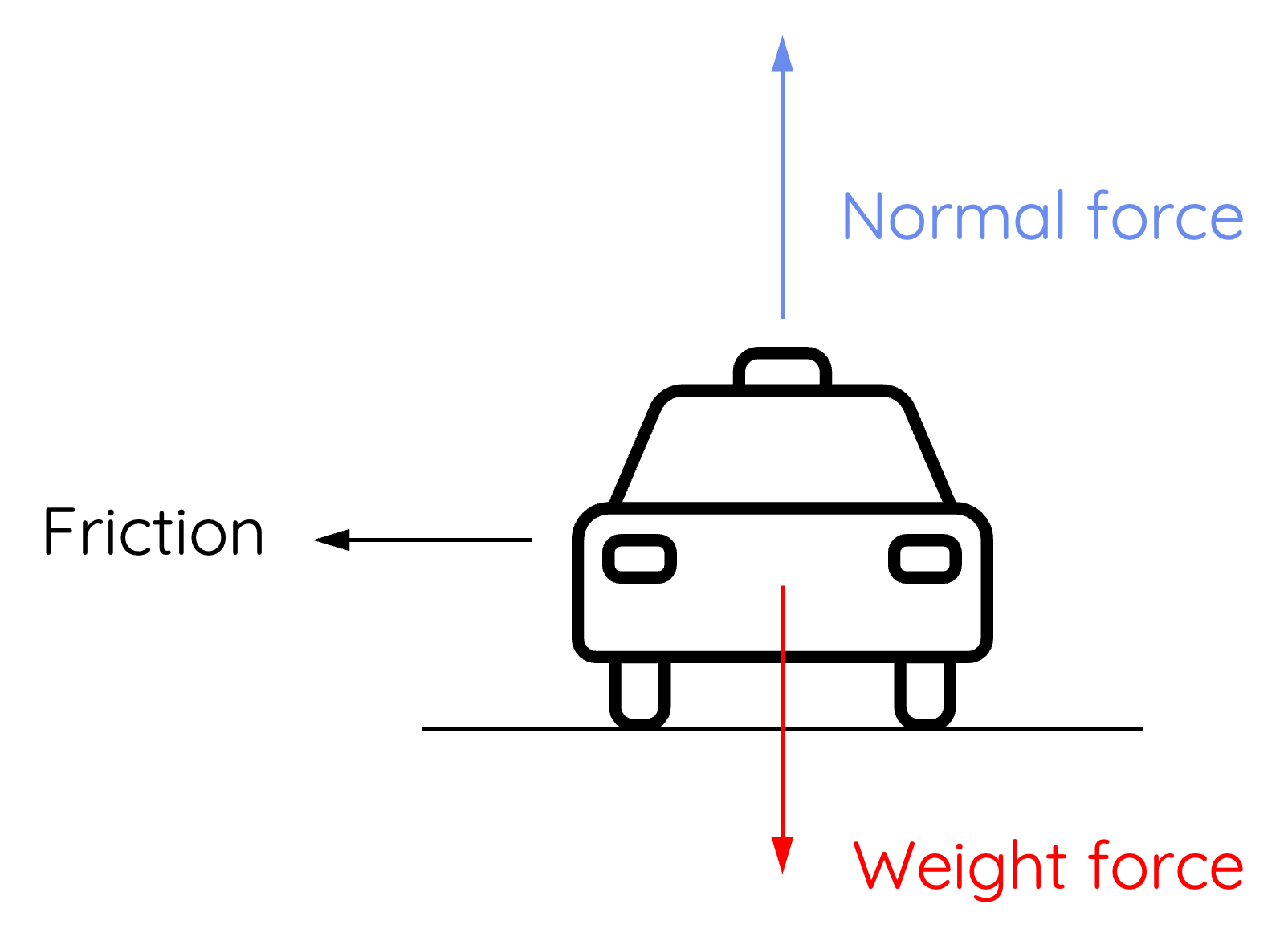# Cars Moving Around Horizontal Circular Bends

This topic is part of the HSC Physics course under the section Circular Motion.

### HSC Physics Syllabus

• analyse the forces acting on an object executing uniform circular motion in a variety of situations, for example:

- cars moving around horizontal circular bends
- a mass on a string
- objects on banked tracks

### Cars around horizontal circular bends

Centripetal force results from friction acting on the object towards ‘centre’ if the bend and orthogonal to the tangential velocity. Drivers or passengers in the car experience acceleration outwards because of inertia (not due to acceleration caused by centripetal force).

For HSC Physics students, understanding the real-world applications of circular motion is paramount. One such everyday occurrence is when a car takes a horizontal turn or bend. In this guide, we’ll break down the forces at work during this process, ensuring clarity and precision for your HSC studies.

### Influencing Factors: Speed, Mass, and Radius

Centripetal force is the net force required to allow for a car to navigate through a horizontal circular bend. It is given by:

$$F_{\text{centripetal}} = \frac{mv^2}{r}$$

Therefore, the magnitude of centripetal force acting on a car in a bend depends on several factors:

1. Speed of the Car: The faster the car, the greater the required centripetal force to keep it in the circular path.
2. Mass of the Car: A heavier car exerts a greater normal force on the road, leading to increased maximum static friction.

### Forces in Action: Friction’s Central Role

In a horizontal turn, there's no banking to assist the car. Instead, the force preventing the car from skidding outwards is friction between the car's tires and the road.

To maintain circular motion, centripetal force is required. In the case of a car in a horizontal bend, this force is provided by friction.

Static Friction the friction that keeps the car from skidding. It acts towards the centre of the circle (the bend) and provides the necessary centripetal force, such that

$$F_{\text{centripetal}} = f_{\text{static}}$$It is important to understand that the magnitude of static friction depends on coefficient of static friction between the surface and the car's tyres, as well as the magnitude of normal force exerted by the surface on the car:

$$f = \mu N$$

### Staying on the Road: The Balance of Forces

For a car to safely navigate a horizontal turn without skidding:

• The necessary centripetal force must be less than or equal to the maximum static friction.
• If a car goes too fast, the required centripetal force will exceed static friction, leading to skidding.

Static friction is related to the normal force exerted by the surface on the car:

$$f = \mu N$$

When a car is moving on a horizontal surface, the net force in the vertical direction is zero. This means the upward normal force balances the downward weight force of the car.

$$N = mg$$

This allows static friction to be expressed in terms of the car's weight force:

$$f = \mu mg$$

Therefore, the centripetal force for a car moving on a horizontal circular bend is given by:

$$F_{\text{centripetal}} = \mu mg$$

Rearranging this equation, we can derive an expression for the maximum velocity of the car around the bend without skidding:

$$\frac{mv^2}{r} = \mu mg$$

$$v = \sqrt{\mu gr}$$

As shown by the equation, the maximum velocity is dependent on several factors:

• Coefficient of static friction between the surface and the car's tyres
• Acceleration due to gravity
• Radius of the circular bend

### Example

A car makes a circular turn of radius 20 m. The coefficient of static friction between the tyres and the road is 0.60.

Calculate the maximum speed at which the car can safely make the turn without sliding.

Solution:

Using the above derivation (you should always include steps of derivation in HSC assessments):

$$v = \sqrt{\mu gr}$$

$$v = \sqrt{(0.60)(9.8)(20)}$$

$$v =10.8 \, m \, s^{-1}$$

Therefore, if the car exceeds this speed, it will lose traction and start skidding off the circular bend.